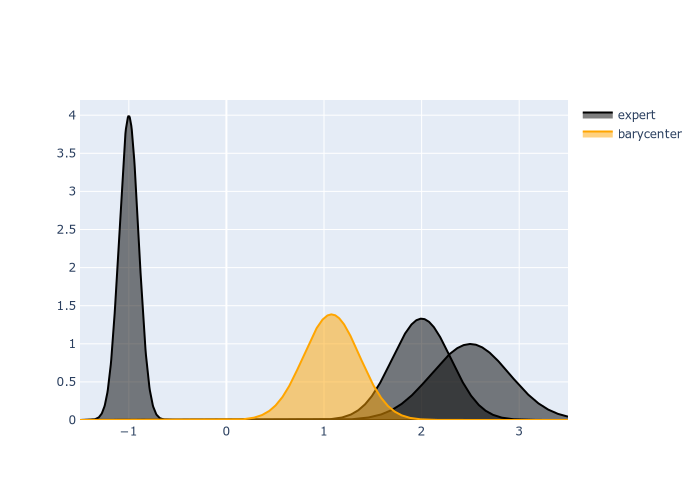# Healing Products of Gaussian Process Experts

Products of Gaussian process experts commonly suffer from poor performance when experts are weak. We propose aggregations and weighting approaches to heal these expert models.

Gaussian processes1 are Bayesian models that allow for predicting while retaining meaningful error bars, but they scale cubically in the size of the training dataset. Approximations have been proposed allowing for improved scaling, including mixture-of-experts and product-of-expert approaches. However, they fall prey to significantly inferior calibration. In our paper2, we propose aggregations and weighting approaches for PoEs that remediate failures of such models and enable better calibrated predictions.

# Products of Gaussian ProcessesFigure 1: Illustration of product-of-GP-expert models. Each expert GP is assigned a local subset of the training data, and aggregation is performed at test time.

Expert models34 approximate a full GP by training $K$ GPs on local subsets of the data, and aggregating using various methods, as illustrated in Figure 1. For PoEs in particular, predictions scale as $O(Km^3)$ where $m$ is the size of the local subset. All PoEs share the same training procedure, consisting of maximizing the joint log-marginal likelihood, while sharing hyperparameters:

$$\color{black}\max_\theta \sum_{k=1}^K\log p(\boldsymbol y^{(k)}|\boldsymbol X^{(k)},\boldsymbol \theta)$$

At test time, the aggregated distributions of PoEs are Gaussians. For instance, the generalize product of GP experts (gPoE)3 has the predictive mean and variance $$\color{black}m_{gpoe}(\boldsymbol x_)= \sigma_{(g)poe}^{2}(\boldsymbol x_)\sum_{j=1}^J\color{red}{\beta_{j}(\boldsymbol x_)} \color{blue}\sigma_{j}^{-2}(\boldsymbol x_)\color{green} m_{j}(\boldsymbol x_),\color{black}$$ $$\sigma_{gpoe}^{-2}(\boldsymbol x_)=\sum_{j=1}^J\color{red}\beta_{j}(\boldsymbol x_)\color{blue}\sigma_{j}^{-2}(\boldsymbol x_)$$

where $\color{red}{\beta_{j}(\boldsymbol x_)}$ is the weight of the $j^{th}$ expert at $\boldsymbol x_$, and $\color{green} m_{j}(\boldsymbol x_)$, $\color{blue}{\sigma_{j}^{-2}(\boldsymbol x_)}$ are the predictive mean and precision of the $j^{th}$ expert at $\boldsymbol x_*$. Methods like the gPoE3 and rBCM4 use confidence measures to weight experts, such as the differential entropy.Figure 2: Expert models trained on synthetic data with 3 points per expert. All models display shortcomings, notably under-/over-confidence and erratic mean predictions.

We propose a new member of the family, arising from the optimal transport theory5, the barycenter of GPs. It is trained in the same way as previously proposed PoEs, and aggregation is performed as follows: $$\color{black}m_{bar}(\boldsymbol x_)= \sum_{j=1}^J\color{red}{\beta_{j}(\boldsymbol x_)} \color{green} m_{j}(\boldsymbol x_),\color{black}$$ $$\sigma_{bar}^{2}(\boldsymbol x_)=\sum_{j=1}^J\color{red}\beta_{j}(\boldsymbol x_)\color{blue}\sigma_{j}^{2}(\boldsymbol x_).$$

However, all such approaches share erratic mean predictions and uncalibrated uncertainty quantification. For instance, we observe in Figure 2 that the PoE is over-confident, the gPoE is over-conservative near the data, and the BCM and rBCM’s predictive means are erratic in the transitioning region.

# Calibrating PoE modelsTemperature: 1 Figure 3: Evolution of the barycenter of GP expert's predictive distribution as T varies. The higher the temperature T, the closer it is pulled to the most confident expert.

Intuitively, the lower the number of points assigned to individual experts, the weaker the experts are overall if the training data associated with these is not dense in the vicinity of test inputs. If weak experts are given weight in the aggregated prediction, uncertainties become uncalibrated and mean predictions erratic. Whilst the gPoE and rBCM allow for confidence-based weighting, we observe erratic and uncalibrated predictions because weight sparsity is not controlled well-enough. We thus propose the following generic weighting approach, motivated by the extensive uncertainty calibration literature, and illustrate it in Figure 3:

$$\color{black} \beta_{j}(\boldsymbol x_)\propto\exp({-T\ \psi_j(\boldsymbol x_)}),\quad \sum_{j=1}^M \beta_j(\boldsymbol x_*) = 1.$$ Mainly, we use softmax weights with a temperature controlling the weight sparsity explicitely. As $T$ grows, only very confident experts will get weight in the decision.Figure 4: Full GP baseline (orange) and expert models (blue) trained with a decreasing number of points per experts (Left to Right), and for different weighting methods: rBCM with differential entropy (Top) and gPoE with the proposed softmax-variance (Bottom).

Figure 4 shows that our weighting proposal softmax-var is significantly more robust to the decrease in the number of points per experts than the differential entropic weighting. This is because we control explicitly weight sparsity, only allowing confident experts to be given weight in the predictions.Figure 5: NLPD of rBCM with differential entropy, and gPoE/Bar with proposed softmax-var weighting. Latter models significantly outperform the former, suggesting better uncertainty calibration.

In Figure 5, we see that on small and large-scale datasets, our weighting proposal often significantly outperforms other weighting proposals in NLPD, while never worsening results. Calibration is thus improved under our proposals.

# Concluding Remarks

• PoE models suffer when weak experts contribute to the overall predictions, leading to
• Erratic mean predictions
• Uncalibrated uncertainties
• We propose a fix, consisting of a tempered softmax weighting scheme, enabling controlling weight sparsity explicitly, remediating such problems across small and large benchmarks
• We also propose a new principled member of the PoE family, the barycenter of GPs that performs on par with the best PoEs across benchmarks

1. C.E. Rasmussen, C.K.I. Williams. Gaussian Processes for Machine Learning. MIT Press. 2006. ↩︎

2. S. Cohen, R. Mbuvha, T. Marwala, M.P. Deisenroth. Healing Products of Gaussian Process Experts. ICML 2020. ↩︎

3. Y. Cao, D.J. Fleet. Generalized Product of Experts for Automatic and Principled Fusion of Gaussian Process Predictions. MN3 Workshop NIPS 2015. ↩︎ ↩︎ ↩︎

4. M.P. Deisenroth, J.W. Ng. Distributed Gaussian Processes. ICML 2015 ↩︎ ↩︎

5. G. Peyré, M. Cuturi. Computational Optimal Transport. Foundations and Trends in Machine Learning. 2018. ↩︎